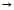# MCQ (Previous Year Questions) - Coordination Compound (Level 2)

## 24 Questions MCQ Test Chemistry for JEE Advanced | MCQ (Previous Year Questions) - Coordination Compound (Level 2)

Description
Attempt MCQ (Previous Year Questions) - Coordination Compound (Level 2) | 24 questions in 48 minutes | Mock test for JEE preparation | Free important questions MCQ to study Chemistry for JEE Advanced for JEE Exam | Download free PDF with solutions
QUESTION: 1

### The pair of compounds having metals in their highest oxidation state is          [JEE-2004]

Solution:

Let oxidation number of Mn be X and that of Cr be Y.

In [MnO4]-- , X + 4(-2) = -1 where -2 is oxidation number of oxygen.

• X = 7 which is the highest for Mn.

Similarly in CrO2Cl2, Y + 2(-2) + 2(-1) = 0.

• Y = 6 which is the highest for Cr

Hence B is correct.

QUESTION: 2

Solution:
QUESTION: 3

### Spin only magnetic moment of the compound Hg[Co(SCN)4] is       [JEE-2004]

Solution:
QUESTION: 4

Which of the following pair is expected to exhibit same colour in solution ?   [JEE-2005]

Solution:
QUESTION: 5

Which type of isomerism is shown by [Co(NH3)4Br2] Cl ?       [JEE-2005]

Solution:
QUESTION: 6

The coordination number of Ni2+ is 4.                    [JEE-2006]

NiCl2 + KCN (excess)A (cyanido complex)

NiCl2 + Conc. KCl (excess)B (chlorido complex)

The IUPAC name of A and B are

Solution:
QUESTION: 7

The coordination number of Ni2+ is 4.                          [JEE-2006]

NiCl2 + KCN (excess)A (cyanido complex)

NiCl2 + Conc. KCl (excess)B (chlorido complex)

Predict the magnetic nature of A and B

Solution:
QUESTION: 8

The coordination number of Ni2+ is 4.                           [JEE-2006]

NiCl2 + KCN (excess)A (cyanido complex)

NiCl2 + Conc. KCl (excess)B (chlorido complex)

The hybridization of A and B are

Solution:
QUESTION: 9

If the bond length of CO bond in carbon monoxides is 1.128 Å, then what is the value of CO bond length in Fe(CO)5 ?           [JEE-2006]

Solution: Because due to synergic bond formation between metal and CO, the bond order of CO decreases.
QUESTION: 10

Among the following metal carbonyls, the C– O bond order is lowest in                    [JEE-2007]

Solution:
QUESTION: 11

Match the complexes in Column I with their properties listed in Column II. Indicate your answer by darkening the appropriate bubbles of the 4 × 4 matrix given in the ORS.        [JEE-2007]

Column I Column - II

(A) [Co(NH3)4(H2O) 2]Cl2 (P) Geometrical isomers

(B) [Pt(NH3)2Cl2] (Q) Paramagnetic

(C) [Co(H2O)5Cl]Cl (R) Diamagnetic

(D) [Ni(H2O)6]Cl2 (S) Metal ion with

+2 oxidation state

Solution:
QUESTION: 12

Among the following, the coloured compound is            [JEE-2008]

Solution:
QUESTION: 13

The IUPAC name of [Ni(NH3)4] [NiCl4] is                        [JEE-2008]

Solution:
QUESTION: 14

Both [Ni(CO)4] and [Ni(CN)4]2_ are diamagnetic. The hybridisations of nickel in these complexes, respectively, are           [JEE-2008]

Solution:
QUESTION: 15

Statement - 1 [Fe(H2O)5 NO]SO4 is paramagnetic.

and

Statement - 2 The Fe in [Fe(H2O)5NO]SO4 has three unpaired electrons.      [JEE-2008]

Solution:
QUESTION: 16

The spin only magnetic moment value (in Bohr magneton units) of Cr(CO)6 is     [JEE-2009]

Solution:
*Multiple options can be correct
QUESTION: 17

The compound(s) that exhibit (s) geometrical isomerism is (are)                                            [JEE-2009]

Solution:
*Multiple options can be correct
QUESTION: 18

The correct structure of ethylenediaminetetraacetic acid (EDTA) is                               [JEE-2010]

Solution:
QUESTION: 19

The ionization isomer of [Cr(H2O)4Cl(NO2)]Cl is                                               [JEE-2010]

Solution:
QUESTION: 20

The complex showing a spin - only magnetic moment of 2.82 B.M. is                                [JEE-2011]

Solution:
QUESTION: 21

Among the following complexes (K _ P), K3[Fe(CN)6] (K), [Co(NH3)6]Cl3 (L), Na3[Co(oxalate)3] (M), [Ni(H2O)6]Cl2(N), K2[Pt(CN)4] (O) and [Zn(H2O)6](NO3) 2 (P) the diamagnetic complexes are                              [JEE-2011]

Solution:
QUESTION: 22

The colour of light absorbed by an aqueous solution of CuSO4 is                          [JEE-2012]

Solution: Since CuSO4 is blue in colour
emitted colour is blue.
But emitted and absorbed colors are complementary colors.
So complementary colour of blue is Orange colour.
QUESTION: 23

As per IUPAC nomenclature, the name of the complex, [Co(H2O)4(NH3) 2]Cl3 is                           [JEE-2012]

Solution:
QUESTION: 24

NiCl2{P(C2H5) 2(C6H5)}2 exhibits temperature dependent magnetic behaviour (paramagnetic / diamagnetic). The coordination geometries of Ni2+ in the paramagnetic and diamagnetic states are respectively.

Solution:Use Code STAYHOME200 and get INR 200 additional OFF Use Coupon Code